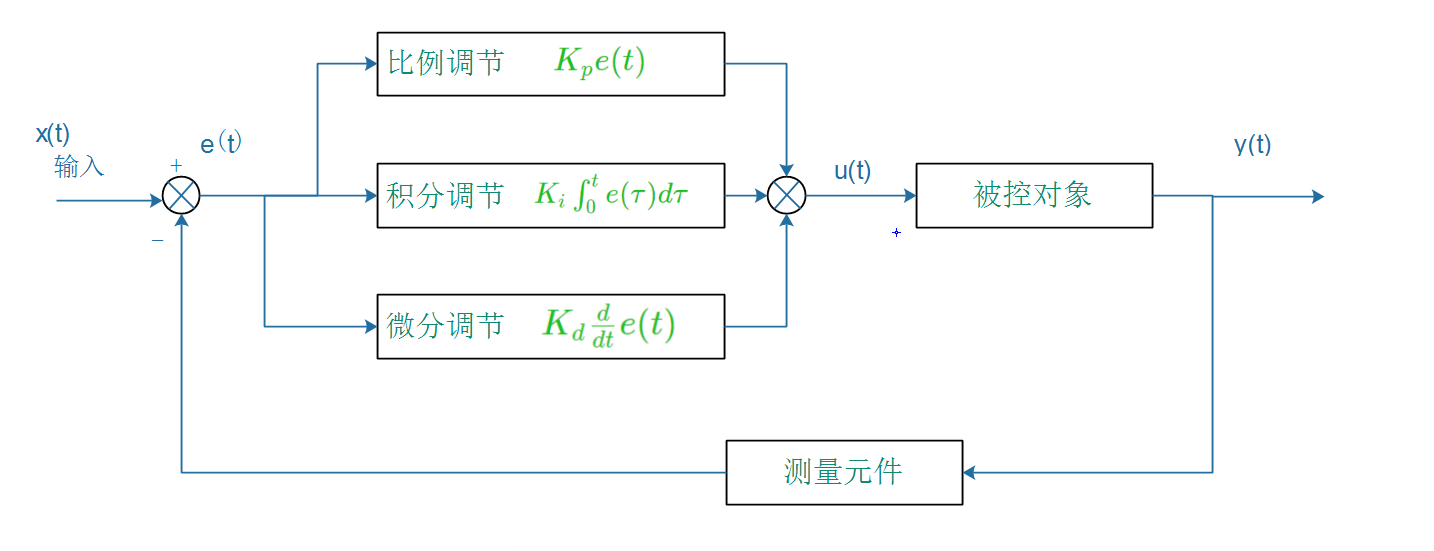##### BOM表：
• Matlab R2017a（博主的）
• Proteus 8.0
• Keil 5

1. PID数学原理分析

### 1. PID数学原理分析

PID控制器，由比例调节（Proportion）、积分调节（Integral）、微分调节（Differential）组成。作用为使用比例、积分、微分这三种调节算法对输入的误差进行处理后，继而输出。

$u(t)=K_{p}e(t)+ K_{i}\int_{0}^{t} e(\tau ) d\tau + K_{d}\frac{d}{dt} e(t)$
$u(t)$: 控制输出
$e(t)$: 误差, 即设定值 - 现在值
$K_{p}$: 比例增益
$K_{i}$: 积分增益
$K_{d}$: 微分增益

PID控制器示意图:$u(t) = K_p(e(t) + \int_0^t\frac{e(t)}{T_i}dt + T_d\frac{de(t)}{dt})$
$T_i$:积分时间
$T_d$:微分时间